Related Articles

# SQL | Conditional Expressions

• Difficulty Level : Medium
• Last Updated : 21 Mar, 2018

Following are Conditional Expressions in SQL

1. The CASE Expression: Let you use IF-THEN-ELSE statements without having to invoke procedures.
In a simple CASE expression, the SQL searches for the first WHEN……THEN pair for which expr is equal to comparison_expr and returns return_expr. If above condition is not satisfied, an ELSE clause exists, the SQL returns else_expr. Otherwise, returns NULL.
We cannot specify literal null for the return_expr and the else_expr. All of the expressions(expr, comparison_expr, return_expr) must be of the same data type.
Syntax:
```CASE expr WHEN comparison_expr1 THEN return_expr1
[WHEN comparison_expr2 THEN return_expr2
.
.
.
WHEN comparison_exprn THEN return_exprn
ELSE else_expr]
END
```

Example:

```Input :
SELECT first_name, dpartment_id, salary,
CASE dpartment_id WHEN 50 THEN 1.5*salary
WHEN 12 THEN 2.0*salary
ELSE salary
END "REVISED SALARY"
FROM Employee;```

Output :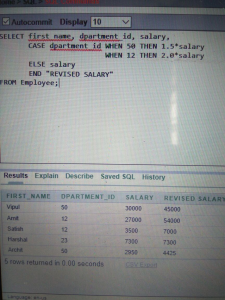Explanation: In above SQL statements, the value of department_id is decoded. If it is 50 then salary is made 1.5 times, if it is 12 then salary is made 2 times, else there is no change in salary.

2. The DECODE Function : Facilitates conditional inquiries by doing the work of a CASE or IF-THEN-ELSE statement.
The DECODE function decodes an expression in a way similar to the IF-THEN-ELSE logic used in various languages. The DECODE function decodes expression after comparing it to each search value. If the expression is the same as search, result is returned.
If the default value is omitted, a null value is returned where a search value does not match any of the result values.
Syntax:

```DECODE(col/expression, search1, result1
[, search2, result2,........,]
[, default])
```
```Input :
SELECT first_name, dpartment_id, salary,
DECODE(dpartment_id, 50, 1.5*salary,
12, 2.0*salary,
salary)
"REVISED SALARY"
FROM Employee;
```

Output :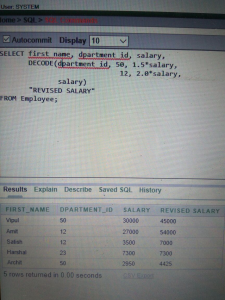Explanation: In above SQL statements, the value of department_id is tested. If it is 50 then salary is made 1.5 times, if it is 12 then salary is made 2 times, else there is no change in salary.

3. COALESCE : Returns the first non-null argument. Null is returned only if all arguments are null. It is often used to substitute a default value for null values when data is retrieved for display.
NOTE: Same as CASE expressions, COALESCE also will not evaluate the arguments to the right of the first non-null argument found.
Syntax:
```COALESCE(value [, ......] )
```
```Input:
SELECT COALESCE(last_name, '- NA -')
from Employee;
```

Output: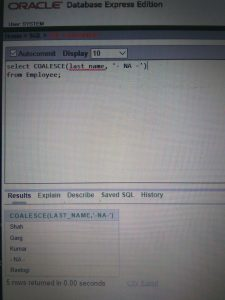Explanation: “- NA -” will be displayed in place where last name is null else respective last names will be shown.

4. GREATEST: Returns the largest value from a list of any number of expressions. Comparison is case sensitive. If datatypes of all the expressions in the list are not same, rest all expressions are converted to the datatype of the first expression for comparison and if this conversion is not possible, SQL will throw an error.
NOTE: Returns null if any expression in the list is null.
Syntax:
```GREATEST(expr1, expr2 [, .....] )
```
• ```Input:
SELECT GREATEST('XYZ', 'xyz')
from dual;
```
```Output:
GREATEST('XYZ', 'xyz')
xyz
```

Explanation: ASCII value of small alphabets is greater.

• ```Input:
SELECT GREATEST('XYZ', null, 'xyz')
from dual;

Output:
GREATEST('XYZ', null, 'xyz')
-```

Explanation: Since null is present hence, null will be shown as output (as mentioned to note in description above).

5. IFNULL: If expr1 is not NULL, returns expr1; otherwise it returns expr2. Returns a numeric or string value, depending on the context in which it is used.
Syntax:
```IFNULL(expr1, expr2)
```
• ```Input:
SELECT IFNULL(1,0)
FROM dual;
```
```Output:
-
1
```

Explanation : Since, no expression is null.

• ```Input:
SELECT IFNULL(NULL,10)
FROM dual;

Output:
--
10
```

Explanation:Since, expr1 is null hence, expr2 is shown.

6. IN: Checks whether a value is present within a set of values and can be used with WHERE, CHECK and creation of views.
NOTE: Same as CASE and COALESCE expressions, IN also will not evaluate the arguments to the right of the first non-null argument found.
Syntax:
```WHERE column IN (x1, x2, x3 [,......] )
```
```Input:
SELECT * from Employee
WHERE dpartment_id IN(50, 12);
```

Output: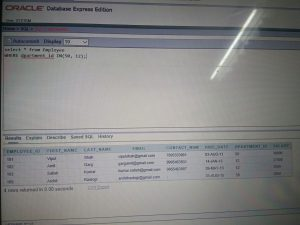Explanation:All data of Employees is shown with department ID 50 or 12.

7. LEAST: Returns the smallest value from a list of any number of expressions. Comparison is case sensitive. If datatypes of all the expressions in the list are not same, rest all expressions are converted to the datatype of the first expression for comparison and if this conversion is not possible, SQL will throw an error.
NOTE: Returns null if any expression in the list is null.

Syntax:

```LEAST(expr1, expr2 [, ......])
```
• ```
strong>Input:
SELECT LEAST('XYZ', 'xyz')
from dual;

Output:
LEAST('XYZ', 'xyz')
XYZ
```

Explanation: ASCII value of capital alphabets is smaller.

• ```
Input:
SELECT LEAST('XYZ', null, 'xyz')
from dual;

Output:
LEAST('XYZ', null, 'xyz')
-
```

Explanation: Since null is present hence, null will be shown as output (as mentioned to note in description above).

8. NULLIF: Returns a null value if value1=value2, otherwise it returns value1.
Syntax:
```NULLIF(value1, value2)
```

Example:

```
Input:
SELECT NULLIF(9995463931, contact_num)
from Employee;
```

Output: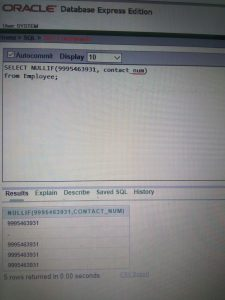Explanation: NULL is displayed for the Employee whose number is matched with the given number. For rest of the Employees value1 is returned.

This article is contributed by akanshgupta. If you like GeeksforGeeks and would like to contribute, you can also write an article using contribute.geeksforgeeks.org or mail your article to contribute@geeksforgeeks.org. See your article appearing on the GeeksforGeeks main page and help other Geeks.